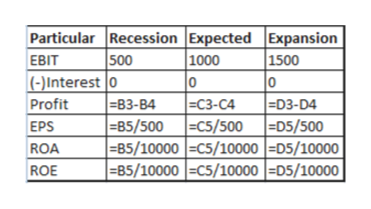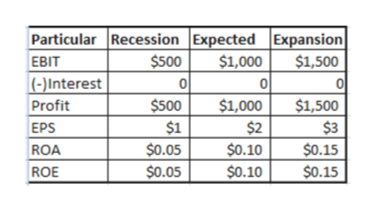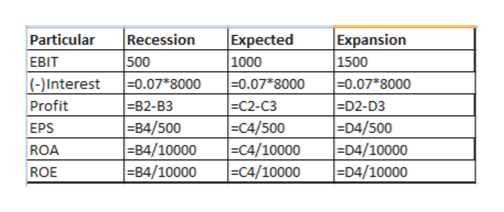# XYZ company has the following expected cash flows for three scenarios that could occur:                         Recession        Expected         Expansion                        (prob. = .2)      (prob. = .5)      (prob. =.3)EBIT               \$10,000           \$20,000          \$30,000MV Assets        ______            ______          ______  (a) Complete the table above if the company is 100% equity financed and the non-levered return on equity is expected to be 12% (b) If the company pays tax at a 30% rate and it wants to recapitalize (debt for equity swap) to save on taxes, what is the most debt the company can add (at a 6% rate) so that it will never go bankrupt under the above scenarios? (Assume the company goes bankrupty if EBIT < Interest owed) (c) Calculate the WACC for the unlevered case and for the result in part (b). (d) What is the market value of the assets if the firm chooses the debt level in part (b)? (e) If further analysis suggests that the following can be used to predict the cash flows of the company:   EBIT = \$1500 x random number chosen from 1 to 20 What is the optimal deb/equity ratio if the company wants to limit the probability of bankruptcy to 5% or less?

Question
428 views
1. XYZ company has the following expected cash flows for three scenarios that could occur:

Recession        Expected         Expansion

(prob. = .2)      (prob. = .5)      (prob. =.3)

EBIT               \$10,000           \$20,000          \$30,000

MV Assets        ______            ______          ______

(a) Complete the table above if the company is 100% equity financed and the non-levered return on equity is expected to be 12%

(b) If the company pays tax at a 30% rate and it wants to recapitalize (debt for equity swap) to save on taxes, what is the most debt the company can add (at a 6% rate) so that it will never go bankrupt under the above scenarios? (Assume the company goes bankrupty if EBIT < Interest owed)

(c) Calculate the WACC for the unlevered case and for the result in part (b).

(d) What is the market value of the assets if the firm chooses the debt level in part (b)?

(e) If further analysis suggests that the following can be used to predict the cash flows of the company:   EBIT = \$1500 x random number chosen from 1 to 20

What is the optimal deb/equity ratio if the company wants to limit the probability of bankruptcy to 5% or less?

check_circle

Step 1

In an levered firm, there is no debt amount therefore no interest amount is payable that is 0. The EPS stands for earning per share deteremined by dividing the net Profit after interest and taxes with the number of equity share holders of the company. In the given case, no tax rate is given therefore it is taken is 0. The ROA stands for return on assets and it it obtained by dividing the net progit with the total average assets of the company. The ROE stands for return on equity and it is ascertained by dividing the net income with the shareholder’s equity amount of the company.help_outlineImage TranscriptioncloseParticular Recession Expected Expansion 1000 0 |ЕC3-С4 | =85/500 C5/500 | =B5/10000 =C5/10000 =D5/10000 |B5/10000 =C5/10000| =D5/10000| 500 (-)Interest 0 83-B4 1500 ЕВIT D3-D4 D5/500 Profit EPS ROA ROE fullscreen
Step 2

Under unlevered firm, the EPS in each scenario recession, expected and expansion is \$1, \$2, \$3 respectively.

The ROA is \$0.05, \$0.10, \$0.15 and ROE is \$0.05, \$0.10 and \$0.15.help_outlineImage TranscriptioncloseParticular Recession Expected Expansion \$500 \$1,500 \$1,000 EBIT (-)Interest Profit \$500 \$1,000 \$1,500 \$1 \$2 \$3 EPS \$0.05 \$0.10 \$0.15 ROA \$0.05 \$0.10 \$0.15 ROE fullscreen
Step 3

In an levered firm, the interest is calcultaed by multiplying the interest rate with the debt amount. The EPS stands for earning per share deteremined by dividing the net Profit after interest and taxes with the number of equity share holders of the company. In the given case, no tax rate is given ther...help_outlineImage TranscriptioncloseRecession 500 0.07 8000 |-B2-вз =84/500 B4/10000 =84/10000 Expected 1000 0.07*8000 ЕC2-СЗ C4/500 =C4/10000 C4/10000 Expansion 1500 =0.07*8000 -D2-D3 =D4/500 D4/10000 |D4/10000 Particular ЕEBIT (-) Interest Profit EPS ROA ROE fullscreen

### Want to see the full answer?

See Solution

#### Want to see this answer and more?

Solutions are written by subject experts who are available 24/7. Questions are typically answered within 1 hour.*

See Solution
*Response times may vary by subject and question.
Tagged in